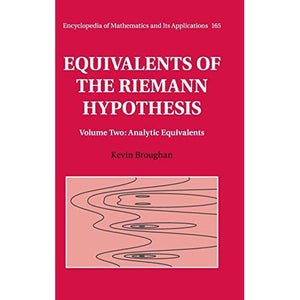># Equivalents of the Riemann Hypothesis: Volume 2, Analytic Equivalents (Encyclopedia of Mathematics and its Applications)

• £56.99
• Save £69

Kevin Broughan
Cambridge University Press, 11/2/2017
EAN 9781107197121, ISBN10: 1107197120

Hardcover, 522 pages, 24.1 x 16.2 x 3.2 cm
Language: English

The Riemann hypothesis (RH) is perhaps the most important outstanding problem in mathematics. This two-volume text presents the main known equivalents to RH using analytic and computational methods. The book is gentle on the reader with definitions repeated, proofs split into logical sections, and graphical descriptions of the relations between different results. It also includes extensive tables, supplementary computational tools, and open problems suitable for research. Accompanying software is free to download. These books will interest mathematicians who wish to update their knowledge, graduate and senior undergraduate students seeking accessible research problems in number theory, and others who want to explore and extend results computationally. Each volume can be read independently. Volume 1 presents classical and modern arithmetic equivalents to RH, with some analytic methods. Volume 2 covers equivalences with a strong analytic orientation, supported by an extensive set of appendices containing fully developed proofs.

1. Introduction
2. Series equivalents
3. Banach and Hilbert space methods
4. The Riemann Xi function
5. The de Bruijn-Newman constant
6. Orthogonal polynomials
7. Cyclotomic polynomials
8. Integral equations
9. Weil's explicit formula, inequality and conjectures
10. Discrete measures
11. Hermitian forms
12. Dirichlet L-functions
13. Smooth numbers
14. Epilogue
Appendix A. Convergence of series
Appendix B. Complex function theory
Appendix C. The Riemann-Stieltjes integral
Appendix D. The Lebesgue integral on R
Appendix E. Fourier transform
Appendix F. The Laplace transform
Appendix G. The Mellin transform
Appendix H. The gamma function
Appendix I. Riemann Zeta function
Appendix J. Banach and Hilbert spaces
Appendix K. Miscellaneous background results
Appendix L. GRHpack mini-manual
References
Index.